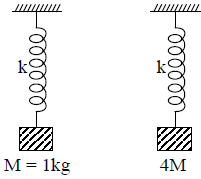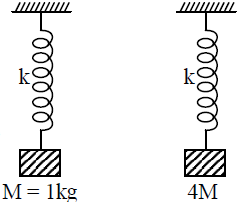Simple hormonic motion
Question

# Two identical springs are fixed at one end and masses M and 4M are suspended at their other ends as shown in figure. They are both stretched down from their mean position and let go simultaneously. If they are in the same phase every 4 seconds, the spring constant k isModerate
Solution

##T2 = 2T1, since these are in phase every 4 second.$\therefore$ T2 = 4 sec.$4=2×2\mathrm{\pi }\sqrt{\frac{1}{\mathrm{k}}}⇒\frac{1}{\sqrt{\mathrm{k}}}=\frac{1}{\mathrm{\pi }}⇒\mathrm{k}={\mathrm{\pi }}^{2}\mathrm{N}/\mathrm{m}$

Get Instant Solutions